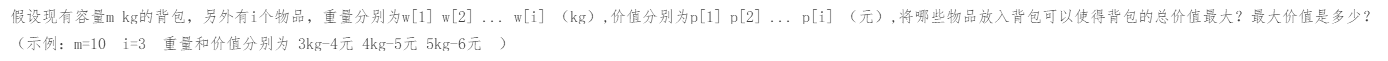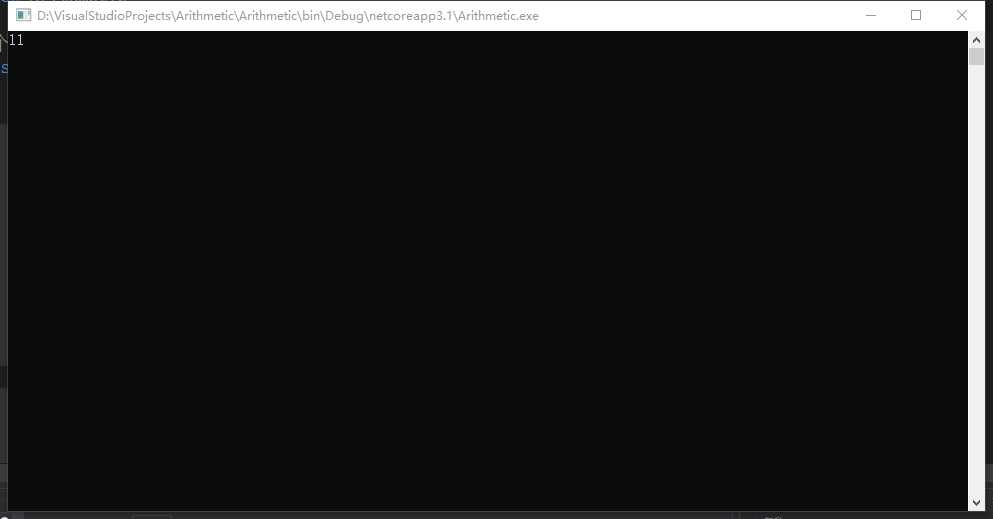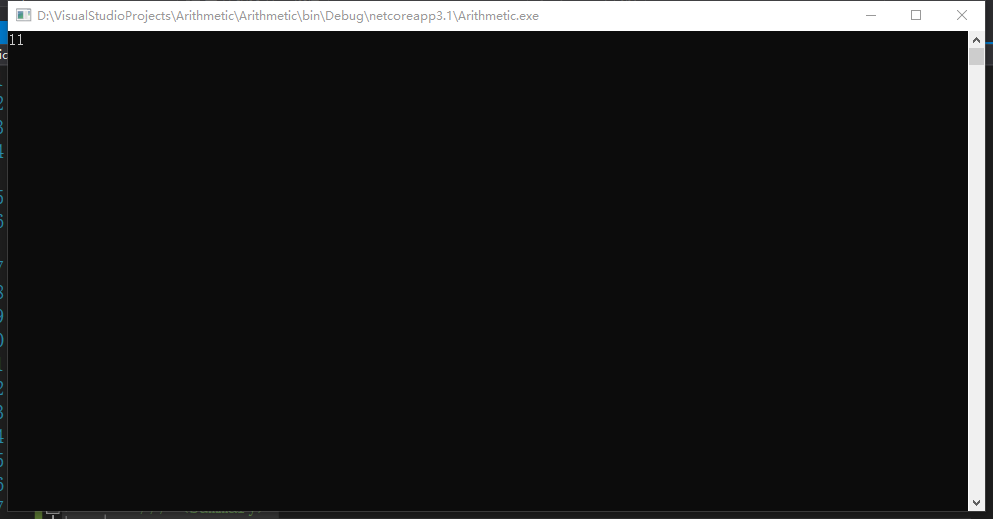### 01背包問題理解動態規劃演算法1.窮舉法

```        static void Main(string[] args)
{
//記錄重量的數組，物品有3種重量，分別是3、4、5
int[] w = { 0, 3, 4, 5 };
//記錄物品價值的數組，和重量數組對應，物品價值分別是4、5、6
int[] p = { 0, 4, 5, 6 };
//調用Exhaustivity函數得到7kg的背包放置物品的最大價值
Console.WriteLine(Exhaustivity(9 , w, p));
Console.ReadKey();
}
/// <summary>
/// 計算給定的mkg背包放置物品的最大價值
/// </summary>
/// <param name="m">給定的物品重量</param>
/// <param name="w">記錄所有物品重量的數組</param>
/// <param name="p">記錄所有物品對應價格的數組</param>
/// <returns>背包中放置物品的最大價值</returns>
public static int Exhaustivity(int m,int[] w,int[] p)
{
//記錄放入物品的最大的價格
int maxPrice = 0;
//有3個物品，外層就循環2的3次方，即i值從0取到7，i的二進位數字最多3位，每一個i的取值的二進位數字都對應3個物品是否放入背包
//如i為6，二進位為110，代表取前兩個物品，不取第3個物品
//又如i為0，二進位為000，代表3個物品均不取；i為1，二進位001，代表只取第3個物品
//通過這種方式窮舉所有物品放入背包的情況，然後判斷每一種情況重量是否滿足要求，再比較價格
for(int i = 0;i < Math.Pow(2,w.Length - 1); i++)
{
//記錄所有被放入背包物品的總重量
int weightTotal = 0;
//記錄所有被放入背包物品的總價格
int priceTotal = 0;
//內層循環負責計算並比較所有物品的總重量和總價格
//內層循環的次數就是物品個數，然後分別判斷當前情況下每個物品是否放入背包，j代表第幾個物品
for(int j = 1;j <= w.Length - 1; j++)
{
//計算當前物品是否放入背包
int result = Get2(i, j);
//在放入背包的情況下，將重量和價格累加到總重量和總價格上
if(result == 1)
{
weightTotal += w[j];
priceTotal += p[j];
}
}
//判斷是否超重，不超重的情況下判斷價格是不是大於最大價格，如果是更新最大價格
if (weightTotal <= m && priceTotal > maxPrice)
maxPrice = priceTotal;
}
//返回最大價格
return maxPrice;
}
/// <summary>
/// 通過按位與運算得到給定物品number在給定放入情況i中是否放入背包
/// </summary>
/// <param name="i">給定的物品放入情況</param>
/// <param name="number">給定的物品編號</param>
/// <returns></returns>
public static int Get2(int i,int number)
{
int A = i;
int B = (int)Math.Pow(2,number - 1);
int result = A & B;
if (result == 0)
return 0;
return 1;
}```2.分治演算法（自上而下分解問題）

這個問題的分治演算法的核心是將物品逐個放入背包，有以下幾種情況：

1）背包沒有剩餘重量了

2）物品太重，無法放入背包

3）物品的編號為0，即所有的物品都試過了

4）物品還可以放入背包

```        static void Main(string[] args)
{
//記錄重量的數組，物品有3種重量，分別是3、4、5
int[] w = { 0, 3, 4, 5 };
//記錄物品價值的數組，和重量數組對應，物品價值分別是4、5、6
int[] p = { 0, 4, 5, 6 };
Console.WriteLine(UpDown(9, w.Length - 1, w, p));
Console.ReadKey();
}
/// <summary>
/// 根據背包剩餘重量和物品編號計算放入物品能獲得最大價值還是不放入物品能獲得最大價值
/// </summary>
/// <param name="m">背包剩餘能裝的重量</param>
/// <param name="i">當前放入背包的物品編號</param>
/// <param name="w">記錄所有物品重量的數組</param>
/// <param name="p">記錄所有物品價格的數組</param>
/// <returns>放入背包的物品的最大價格</returns>
public static int UpDown(int m,int i,int[] w,int[] p)
{
//如果背包還能裝的重量為0或者放入的物品編號為0，價格自然都是0
if (i == 0 || m == 0) return 0;
//將第i個物品放入背包，但是物品放入後超重，這種情況下就不放入這個物品，看下一個物品是否能放入背包（物品編號-1）
if(w[i] > m)
return UpDown(m,i - 1, w, p);
//在第i個物品能放入背包的情況下需要計算比較價格
else
{
//計算並記錄將物品放入背包的情況下的最大價格（遞歸物品編號-1，從背包剩餘重量中扣除當前物品重量，由於當前物品放入背包，背包中物品價格加上當前物品價格）
int maxValue1 = UpDown(m - w[i], i - 1, w, p) + p[i];
//計算並記錄不將物品放入背包的情況下的最大價格（遞歸物品編號-1，但是由於沒有放入物品，背包剩餘重量不變）
int maxValue2 = UpDown(m, i - 1, w, p);
//比較並取其中價格最大的那個
if (maxValue1 > maxValue2)
return maxValue1;
return maxValue2;
}
}```3.改進分治演算法，使用數組記錄已經運算過的問題結果

```        //定義一個規則二維數組記錄結果，行數對應背包重量，列數對應放入的物品個數，值對應這種情況背包中的最大重量
public static int[,] result = new int[11, 4];
static void Main(string[] args)
{
int[] w = { 0, 3, 4, 5 };
int[] p = { 0, 4, 5, 6 };
Console.WriteLine(UpDown(9, w.Length - 1, w, p));
Console.ReadKey();
}
public static int UpDown(int m,int i,int[] w,int[] p)
{
if (i == 0 || m == 0) return 0;
//判斷這種情況是不是已經計算過了，計算過了就直接返回
if (result[m, i] != 0)
return result[m, i];
//在計算完成後需要將計算結果存儲起來
if(w[i] > m)
return result[m,i] = UpDown(m,i - 1, w, p);
else
{
int maxValue1 = UpDown(m - w[i], i - 1, w, p) + p[i];
int maxValue2 = UpDown(m, i - 1, w, p);
if (maxValue1 > maxValue2)
return result[m, i] = maxValue1;
return result[m, i] = maxValue2;
}
}```

4.動態規劃演算法（自下而上計算問題）

```        static void Main(string[] args)
{
int[] w = { 0, 3, 4, 5 };
int[] p = { 0, 4, 5, 6 };
Console.WriteLine(BottomUp(9, w.Length - 1, w, p));
Console.ReadKey();
}
//記錄運算結果的數組，行數代表背包重量，列數代表物品編號
public static int[,] result = new int[11, 4];
/// <summary>
/// 計算指定重量m的背包种放置前i個物品放置的最大價值
/// </summary>
/// <param name="m">背包重量</param>
/// <param name="i">物品編號</param>
/// <param name="w">記錄所有物品重量的數組</param>
/// <param name="p">記錄所有物品價格的數組</param>
/// <returns></returns>
public static int BottomUp(int m,int i,int[] w,int[] p)
{
//外層循環從1到m，背包重量從小到大
for(int tempM = 1;tempM <= m; tempM++)
{
//內層循環從1到i，物品個數從1到i
for(int tempI = 1;tempI <= i;tempI++)
{
//如果已經計算過，不用再計算了
if (result[tempM, tempI] != 0) continue;
//如果當前物品重量超過背包剩餘可裝重量，不放入物品，將物品編號減一
if (w[tempI] > tempM)
result[tempM, tempI] = result[tempM, tempI - 1];
//當前放置的物品重量不超過背包剩餘可裝重量，說明物品能放入背包
//物品不一定放入背包最後的總價值最大，所以需要比較放入和不放入的情況得到的價格哪種更大
else
{
int maxValue1 = result[tempM - w[tempI], tempI - 1] + p[tempI];
int maxValue2 = result[tempM, tempI - 1];
result[tempM, tempI] = maxValue1 > maxValue2 ? maxValue1 : maxValue2;
}
}
}
//自下而上計算完成後，返回重量為m的背包放入i個物品的最大價格即可
return result[m, i];
}```